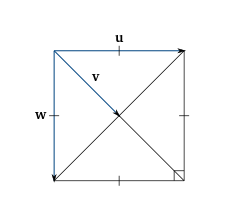Dot Product

(requires JavaScript)

1. Which of the following expression are meaningful? Which are meaningless? Explain.
1. $\left(\mathbf{a}•\mathbf{b}\right)•\mathbf{c}$
2. $\left(\mathbf{a}•\mathbf{b}\right)\mathbf{c}$
3. $\left|\mathbf{a}\right|\left(\mathbf{b}•\mathbf{c}\right)$
4. $\mathbf{a}•\left(\mathbf{b}+\mathbf{c}\right)$
5. $\mathbf{a}•\mathbf{b}+\mathbf{c}$
6. $\left|\mathbf{a}\right|•\left(\mathbf{b}+\mathbf{c}\right)$
b, c, and d are meaningful.
2. Find the dot product of two vectors if their lengths are $6$ and $\frac{1}{3}$ and the angle between them is $\frac{\pi }{4}$.
3. Find $\mathbf{a}•\mathbf{b}$ if $\mathbf{a}=⟨4,1,\frac{1}{4}⟩$, $\mathbf{b}=⟨6,-3,-8⟩$.
$19$
4. Find $\mathbf{a}•\mathbf{b}$ if $\mathbf{a}=4\mathbf{j}-3\mathbf{k}$ and $\mathbf{b}=2\mathbf{i}+4\mathbf{j}+6\mathbf{k}$.
5. If $\mathbf{u}$ is a unit vector, find $\mathbf{u}•\mathbf{v}$ and $\mathbf{u}•\mathbf{w}$.From the figure, $\left|\mathbf{u}\right|=\left|\mathbf{w}\right|=1$, and $\left|\mathbf{v}\right|=\frac{1}{\sqrt{2}}$. The angle between $\mathbf{u}$ and $\mathbf{w}$ is $\pi /2$, and the angle between $\mathbf{u}$ and $\mathbf{v}$ is $\pi /4$. So we can write

$\mathbf{u}•\mathbf{v}=\left|\mathbf{u}\right|\left|\mathbf{v}\right|\mathrm{cos}\left(\pi /4\right)=\frac{1}{2}$

and

$\mathbf{u}•\mathbf{w}=\left|\mathbf{u}\right|\left|\mathbf{w}\right|\mathrm{cos}\left(\pi /2\right)=0$

6. Find the angle between vectors $\mathbf{a}=\mathbf{j}+\mathbf{k}$ and $\mathbf{b}=\mathbf{i}+2\mathbf{j}-3\mathbf{k}$.
${\mathrm{cos}}^{-1}\left(\frac{-1}{2\sqrt{7}}\right)\approx 101°$
7. Determine whether given vectors are orthogonal, parallel, or neither.
1. $\mathbf{a}=⟨-5,3,7⟩$, $\mathbf{b}=⟨6,-8,2⟩$.
2. $\mathbf{a}=⟨4,6⟩$, $\mathbf{b}=⟨-3,2⟩$.
3. $\mathbf{a}=-\mathbf{i}+2\mathbf{j}+5\mathbf{k}$, $\mathbf{b}=3\mathbf{i}+4\mathbf{j}-\mathbf{k}$.
4. $\mathbf{a}=2\mathbf{i}+6\mathbf{j}-4\mathbf{k}$, $\mathbf{b}=-3\mathbf{i}-9\mathbf{j}+6\mathbf{k}$.
Neither, orthogonal, orthogonal, parallel.
8. Find two unit vectors that make an angle of $60°$ with $\mathbf{v}=⟨3,4⟩$.
9. Find the scalar and vector projections of $\mathbf{b}$ onto $\mathbf{a}$, where $\mathbf{a}=\mathbf{i}+\mathbf{j}+\mathbf{k}$ and $\mathbf{b}=\mathbf{i}-\mathbf{j}+\mathbf{k}$.

${\mathrm{comp}}_{\mathbf{a}}\mathbf{b}=\frac{1}{\sqrt{3}}$,

${\mathrm{proj}}_{\mathbf{a}}\mathbf{b}=\frac{1}{3}⟨1,1,1⟩$.

10. Suppose that $\mathbf{a}$ and $\mathbf{b}$ are non-zero vectors. Under what circumstances is
1. ${\mathrm{comp}}_{\mathbf{a}}\mathbf{b}={\mathrm{comp}}_{\mathbf{b}}\mathbf{a}$
2. ${\mathrm{proj}}_{\mathbf{a}}\mathbf{b}={\mathrm{proj}}_{\mathbf{b}}\mathbf{a}$
11. An intelligent robot exerts a horizontal force of $25$ lb on a crate as it pushes it up a ramp that is $10$ feet long and inclined at an angle of $20°$ above the horizontal. Find the work done on the box.
$250\mathrm{cos}\left(20°\right)\approx 235$ ft-lb.
12. Find the angle between a diagonal of a cube and one of its edges.
${\mathrm{cos}}^{-1}\left(\frac{1}{\sqrt{3}}\right)\approx 55°$